Next: The Crank-Nicholson scheme Up: The wave equation Previous: The 1-d advection equation

## The Lax scheme

The instability in the differencing scheme (237) can be fixed by replacing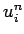on the right-hand side by the spatial average of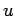taken over the neighbouring grid points. Thus, we obtain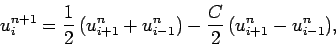(240)

which is known as the Lax scheme. A von Neumann stability analysis of the Lax scheme yields the following expression for the amplification factor: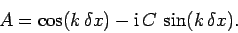(241)

Now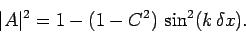(242)

It follows that the Lax scheme is unconditionally stable (i.e.,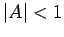for all), provided that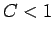. From the definition of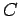, the inequalitycan also be written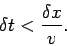(243)

This is the famous Courant-Friedrichs-Lewy (or CFL) stability criterion. In fact, all stable explicit differencing schemes for solving the advection equation are subject to the CFL constraint, which determines the maximum allowable time-step.

Listed below is a routine which solves the 1-d advection equation via the Lax method.

// Lax1D.cpp

// Function to evolve advection equation in 1-d:

//  du / dt + v du / dx = 0  for  xl <= x <= xh

//  u = 0  at x=xl and x=xh

// Array u assumed to be of extent N+2.

// Now, ith element of array corresponds to

//  x_i = xl + i * dx    i=0,N+1

// Here, dx = (xh - xl) / (N+1) is grid spacing.

// Function evolves u by single time-step.

// C = v dt / dx, where dt is time-step.

// Uses Lax scheme.

#include <blitz/array.h>

using namespace blitz;

void Lax1D (Array<double,1>& u, double C)
{
// Set N. Declare local array.
int N = u.extent(0) - 2;
Array<double,1> u0(N+2);

// Evolve u
u0 = u;
for (int i = 1; i <= N; i++)
u(i) = 0.5 * (u0(i+1) + u0(i-1)) - 0.5 * C * (u0(i+1) - u0(i-1));

// Set boundary conditions
u(0) = 0.;
u(N+1) = 0.;
}


Figure 75 shows an example calculation which uses the above routine to advect a Gaussian pulse. The initial condition is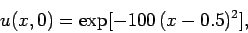(244)

and the calculation is performed with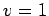,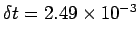, and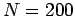. Furthermore,and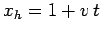. Note that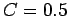for these parameters. It can be seen that the pulse is advected at the correct speed: i.e., the pulse appears approximately stationary when plotted versus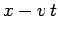. Unfortunately, the pulse does not remain the same shape (as it should). Instead, the pulse becomes gradually lower and wider as it propagates, and eventually diffuses away entirely.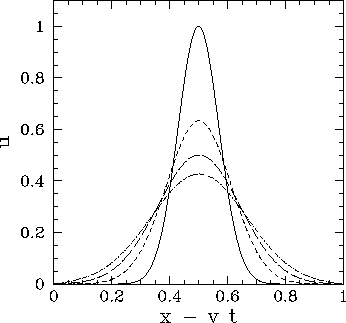It is clear, from the above calculation, that the Lax scheme introduces a spurious dispersion effect into the advection problem. We can understand the origin of this effect by attempting a Fourier solution,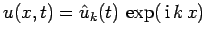, of Eq. (234). We easily obtain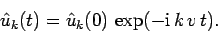(245)

Note that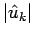is constant in time for all values of. In other words, the amplitudes of the Fourier harmonics of a true solution of the advection equation remain constant in time--it is the phases of the harmonics which evolve. Let us now examine Eq. (242). It can be seen that, provided the CFL conditionis satisfied, the magnitude of the amplification factor,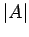, is less than unity for all Fourier harmonics. In other words, the Lax differencing scheme causes the Fourier harmonics to decay in time. It is this unphysical attenuation of the Fourier harmonics which gives rise to the strong dispersion effect illustrated in Fig. 75.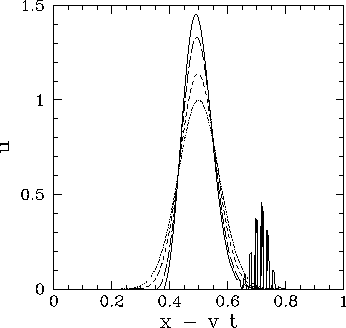Figure 76 shows a calculation made using the Lax scheme in which the CFL condition is violated. This calculation is identical to the one discussed previously, except that the time-step has been increased to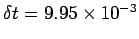, yielding a CFL parameter,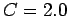, which exceeds unity. It can be seen that the pulse grows in amplitude, and eventually starts to break up due to a short-wavelength instability.Next: The Crank-Nicholson scheme Up: The wave equation Previous: The 1-d advection equation
Richard Fitzpatrick 2006-03-29